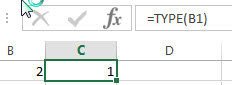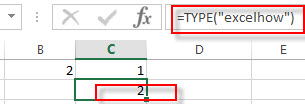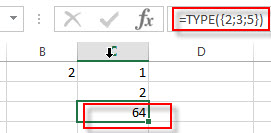## Excel TYPE Function

This post will guide you how to use Excel TYPE function with syntax and examples in Microsoft excel.

### Description

The Excel TYPE function returns the type of a value. So you can use the TYPE function to return an integer that represents the value’s type in Excel. For example, if the value is a number, The TYPE function returns 1, and if the value is a text value, then the function will return 2.

The TYPE function is a build-in function in Microsoft Excel and it is categorized as an Information Function.

The TYPE function is available in Excel 2016, Excel 2013, Excel 2010, Excel 2007, Excel 2003, Excel XP, Excel 2000, Excel 2011 for Mac.

### Syntax

The syntax of the TYPE function is as below:

`= TYPE (value)`

Where the TYPE function argument is:

• Value -This is a required argument. The value that you want to get the type. it can be a number, text, logical value, etc.
 If value is TYPE returns Number 1 Text 2 Logical value 4 Error value 16 Array 64

### Excel TYPE Function Example

The below examples will show you how to use Excel TYPE Function to get the type of a value.

#1 to get the type of the value in cell B1, using the following formula:

=TYPE(B1)#2 to get the type of the value “excelhow”, which is text, using the following formula:

= TYPE(“excelhow”)3# to get the type of an array constant {2;3;5}, using the following formula:

= TYPE ({2;3;5})